Next: The Scenario Machine: Operational Up: Evolution of Magnetic Rotators Previous: Ejector-propeller hysteresis

### E-P transitions for different orbits

Orbital motion of the rotator around the normal companion in an eccentric binary draws a horizontal line on the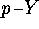diagram, with the beginning at a point corresponding to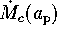, and the end at a point corresponding to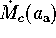(hereand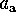are the periasrton and apastron distances, respectively). The length of this segment is determined by the eccentricity. Since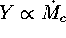, the rotator moves along this segment from left to right and back as it revolves from the apastron to the periastron. At each successive orbital period, this line slowly drifts up to larger periods. The evolution of this system is thus determined by the order the critical lines on the diagram are crossed by this ``line''. It is seen from thediagram that the regions with and without hysteresis are separated by a certain value of the parameter. Since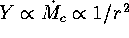, four different situations are possible depending on the relationship of the binary orbital separation a with critical value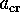, corresponding to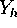(Osminkin and Prokhorov, 1995; see FigureĀ13 and corresponding cases (a)-(d) below).

Figure 13: Possible variants of the ejector-propeller transitions of a NS in an eccentric binary system. The NS rotational period spins downĀ from left to right. Four cases (a-d) correspond to different parameters of binary systems: eccentricity, semi-axis and stellar windĀ parameters as defined in the text. The hatched parts of the ellipses correspond to propeller regime.Ā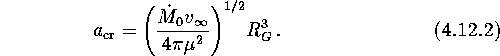1.In this case no hysteresis occurs and transitions E--P and the reverse take place in symmetrical points of the orbit. The rotator passes the following sequence of stages:. Here EP means a mixed state of the rotator at which it is in the ejector state during one part of the orbit, and in the propeller state during the rest of the orbital cycle.
2.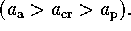In this case, the hysteresis occurs at the beginning of the mixed EP-state (state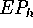), but as the rotator slows down the hysteresis gradually decays and disappears. E-P transition for this system is3.. The hysteresis is possible in principle, but the shape of the transition depends on the eccentricity. Suppose a pulsar has spun down so much that the first transition from the ejector to the propeller occurred at the periastron. If the eccentricity were small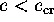, (do not confuse thiswith the critical eccentricity introduced in the previous section) the reverse transition to the ejector state would not occur even at the apastron, and the evolutionary path would be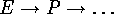.
4. If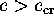, the track is. It should be noted that just after the first EP transition (as well as before the last), the system spends a finite time in the E and P states at every revolution.

The value ofcan be expressed through the orbital parameters asTo conclude, we note that the hysteresis during the ejector-propeller transition may be possible for single radiopulsars also.Ā For example, when the pulsar moves through a dense cloud of interstellar plasma, the pulses can be absorbed. The radiopulsar turns on again when it comes out from the cloud. The hysteresis amplitude for single pulsars can be high enough because of small relative velocities of the interstellar gas and the pulsar, so thatNext: The Scenario Machine: Operational Up: Evolution of Magnetic Rotators Previous: Ejector-propeller hysteresis

Mike E. Prokhorov
Sat Feb 22 18:38:13 MSK 1997